# Question Solved1 AnswerPart J - Analysis of the results Crossing over occurs more frequently between genes separated by greater physical distance. The frequency of cross-over events between pairs of genes can be used to place the genes in sequence on a chromosome, developing a "map" that shows the relative location of genes on a chromosome. A map unit is defined as a crossover frequency of 1% and is a relative measure of the distance between two genes or a gone and the centromero. The greater the number of crossovers, the greater the map distance, When counting asci in the lab, scan the slide on low power until you find a typical starburst ascocarp with asci, then use high power and very dim light to make your observations. You will need to count at least 50 asci to obtain reliable data Study the following small section of a slide of Sordaria to determine if crossing over has occurred in the asci designated with an X. If the ascospores are arranged as 4 tan 4 black, count the ascus as "No crossing over." If the arrangement of ascospores is in any other combination, count it as 'Crossing over Keep a tally of the number of asci showing crossing over and the number that show no crossing over. You will use this data to calculate map distance 0001 090 A Submit Previous Answers Correct If an ascus has a 4:4 spore arrangement, no crossing over has occurred; there are five marked ascl showing this. If an ascus has any other arrangement, crossing over has occurred: six marked asci show crossing over. The number of ascl showing crossing over/total number of asci counted 100 % crossovers. So 6/11 100 = 55% crossovers. LabBench: Cell Division--Mitosis and Meiosis 1 of 1 Part K. Using the frequency of crossing over to map genes From the crossover data you gather for Sordaria, you will be able to calculate the map distance between the gene for ascospore color and the centromere, Centromere Allele for ascospore color Distance of the allele for ascospore color from the centromere To find the number of map units, you divide the percent of crossovers by two. Why divide by two? Each crossover produces two ascospores like the parents and two spores that are a result of the crossover. Thus, to determine the number of crossovers, you must divide the percentage of crossovers by two since only half the spores in each ascus result from crossing over Using the data from the question above, calculate the map distance between the gene for ascospore color and the centromere. 31.8 map units Submit Previous Answers Request Answer 1 Cycle Lab Bench: Cell Division--Mitosis and Meiosis How many asci marked with an X show no evidence of crossing over? 5 7 How many asci marked with an X show evidence of crossing over? 6 3 What is the total number of asci marked with an X? 11 45 What is the percent of crossovers? 55 % 12 10 4 X Х X Dhooo Х -Х X- 4 od coob Х oooooo oooood coccocco X х X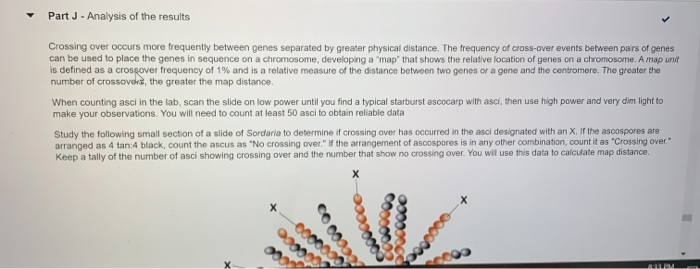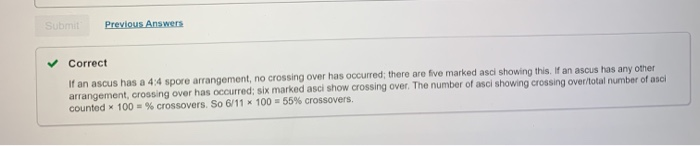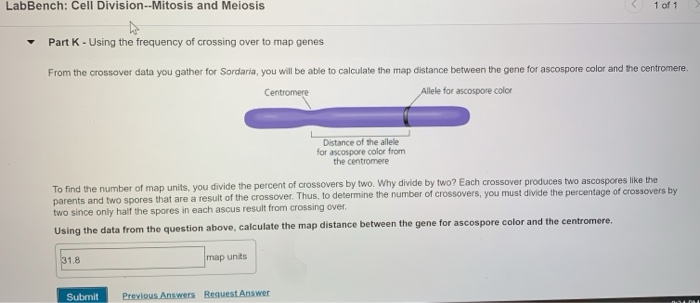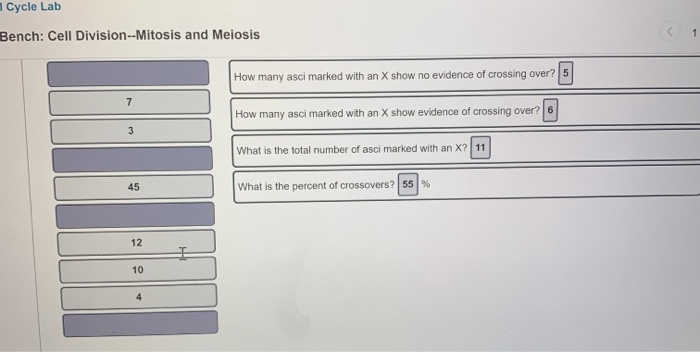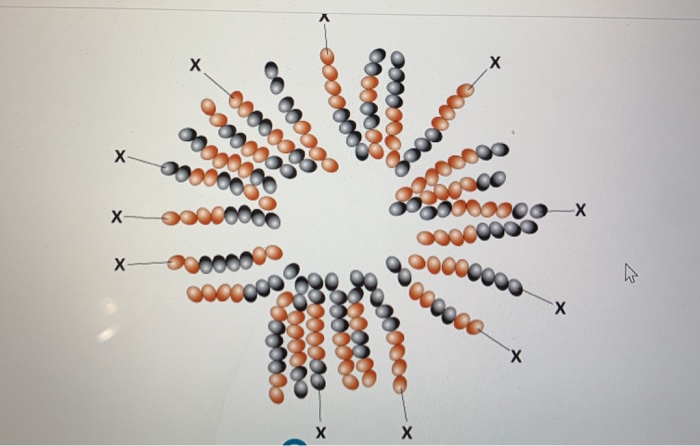Transcribed Image Text: Part J - Analysis of the results Crossing over occurs more frequently between genes separated by greater physical distance. The frequency of cross-over events between pairs of genes can be used to place the genes in sequence on a chromosome, developing a "map" that shows the relative location of genes on a chromosome. A map unit is defined as a crossover frequency of 1% and is a relative measure of the distance between two genes or a gone and the centromero. The greater the number of crossovers, the greater the map distance, When counting asci in the lab, scan the slide on low power until you find a typical starburst ascocarp with asci, then use high power and very dim light to make your observations. You will need to count at least 50 asci to obtain reliable data Study the following small section of a slide of Sordaria to determine if crossing over has occurred in the asci designated with an X. If the ascospores are arranged as 4 tan 4 black, count the ascus as "No crossing over." If the arrangement of ascospores is in any other combination, count it as 'Crossing over Keep a tally of the number of asci showing crossing over and the number that show no crossing over. You will use this data to calculate map distance 0001 090 A Submit Previous Answers Correct If an ascus has a 4:4 spore arrangement, no crossing over has occurred; there are five marked ascl showing this. If an ascus has any other arrangement, crossing over has occurred: six marked asci show crossing over. The number of ascl showing crossing over/total number of asci counted 100 % crossovers. So 6/11 100 = 55% crossovers. LabBench: Cell Division--Mitosis and Meiosis 1 of 1 Part K. Using the frequency of crossing over to map genes From the crossover data you gather for Sordaria, you will be able to calculate the map distance between the gene for ascospore color and the centromere, Centromere Allele for ascospore color Distance of the allele for ascospore color from the centromere To find the number of map units, you divide the percent of crossovers by two. Why divide by two? Each crossover produces two ascospores like the parents and two spores that are a result of the crossover. Thus, to determine the number of crossovers, you must divide the percentage of crossovers by two since only half the spores in each ascus result from crossing over Using the data from the question above, calculate the map distance between the gene for ascospore color and the centromere. 31.8 map units Submit Previous Answers Request Answer 1 Cycle Lab Bench: Cell Division--Mitosis and Meiosis How many asci marked with an X show no evidence of crossing over? 5 7 How many asci marked with an X show evidence of crossing over? 6 3 What is the total number of asci marked with an X? 11 45 What is the percent of crossovers? 55 % 12 10 4 X Х X Dhooo Х -Х X- 4 od coob Х oooooo oooood coccocco X х X
More
Transcribed Image Text: Part J - Analysis of the results Crossing over occurs more frequently between genes separated by greater physical distance. The frequency of cross-over events between pairs of genes can be used to place the genes in sequence on a chromosome, developing a "map" that shows the relative location of genes on a chromosome. A map unit is defined as a crossover frequency of 1% and is a relative measure of the distance between two genes or a gone and the centromero. The greater the number of crossovers, the greater the map distance, When counting asci in the lab, scan the slide on low power until you find a typical starburst ascocarp with asci, then use high power and very dim light to make your observations. You will need to count at least 50 asci to obtain reliable data Study the following small section of a slide of Sordaria to determine if crossing over has occurred in the asci designated with an X. If the ascospores are arranged as 4 tan 4 black, count the ascus as "No crossing over." If the arrangement of ascospores is in any other combination, count it as 'Crossing over Keep a tally of the number of asci showing crossing over and the number that show no crossing over. You will use this data to calculate map distance 0001 090 A Submit Previous Answers Correct If an ascus has a 4:4 spore arrangement, no crossing over has occurred; there are five marked ascl showing this. If an ascus has any other arrangement, crossing over has occurred: six marked asci show crossing over. The number of ascl showing crossing over/total number of asci counted 100 % crossovers. So 6/11 100 = 55% crossovers. LabBench: Cell Division--Mitosis and Meiosis 1 of 1 Part K. Using the frequency of crossing over to map genes From the crossover data you gather for Sordaria, you will be able to calculate the map distance between the gene for ascospore color and the centromere, Centromere Allele for ascospore color Distance of the allele for ascospore color from the centromere To find the number of map units, you divide the percent of crossovers by two. Why divide by two? Each crossover produces two ascospores like the parents and two spores that are a result of the crossover. Thus, to determine the number of crossovers, you must divide the percentage of crossovers by two since only half the spores in each ascus result from crossing over Using the data from the question above, calculate the map distance between the gene for ascospore color and the centromere. 31.8 map units Submit Previous Answers Request Answer 1 Cycle Lab Bench: Cell Division--Mitosis and Meiosis How many asci marked with an X show no evidence of crossing over? 5 7 How many asci marked with an X show evidence of crossing over? 6 3 What is the total number of asci marked with an X? 11 45 What is the percent of crossovers? 55 % 12 10 4 X Х X Dhooo Х -Х X- 4 od coob Х oooooo oooood coccocco X х X